Mathematics Commons™

16,953 Full-Text Articles 16,266 Authors 4,137,002 Downloads277 Institutions

All Articles in Mathematics

16,953 full-text articles. Page 483 of 491.

2010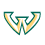Wayne State University

Asymptotic Properties Of Markov Modulated Sequences With Fast And Slow Time Scales, Son Luu Nguyen

Wayne State University Dissertations

In this dissertation we investigate asymptotic properties of Markov modulated random processes having two-time scales. The model contains a number of mixing sequences modulated by a switching process that is a discrete-time Markov chain. The motivation of our study stems from applications in manufacturing systems, communication networks, and economic systems, in which regime-switching models are used.

This thesis focuses on asymptotic properties of the Markov modulated processes under suitable scaling. Our main effort focuses on obtaining weak convergence and strong approximation results.

Morphing Of Triangular Meshes In Shape Space, 2010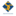NRC Institute for Information Technology

Morphing Of Triangular Meshes In Shape Space, Stefanie Wuhrer, Prosenjit Bose, Chang Shu, Joseph O'Rourke, Alan Brunton

Computer Science: Faculty Publications

We present a novel approach to morph between two isometric poses of the same non-rigid object given as triangular meshes. We model the morphs as linear interpolations in a suitable shape space S. For triangulated 3D polygons, we prove that interpolating linearly in this shape space corresponds to the most isometric morph in R3 . We then extend this shape space to arbitrary triangulations in 3D using a heuristic approach and show the practical use of the approach using experiments. Furthermore, we discuss a modified shape space that is useful for isometric skeleton morphing. All of the newly presented approaches ...

2010St. John Fisher College

Matching Functions And Graphs At Multiple Levels Of Bloom’S Revised Taxonomy, Kris H. Green

Mathematical and Computing Sciences Faculty/Staff Publications

This paper illustrates the power of Bloom's revised taxonomy for teaching, learning and assessing  in aligning our curriculum expectations and our assessment tools in multivariable calculus. The particular assessment tool considered involves a common matching problem to evaluate students' abilities to think about functions from graphical and formulaic representations. Through this analysis we gain additional understanding of why students may have difficulty in performing well on certain activities.

If Mowat And Davis Are Correct, Then Teaching Is Hard: A Response To Elizabeth Mowat & Brent Davis, 2010St. John Fisher College

If Mowat And Davis Are Correct, Then Teaching Is Hard: A Response To Elizabeth Mowat & Brent Davis, Kris H. Green, Bernard P. Ricca

Mathematical and Computing Sciences Faculty/Staff Publications

In lieu of an abstract, below is the article's first paragraph.

Mowat & Davis (this issue) present a model of learning mathematics that relies heavily on ideas from network (or graph) theory. The important questions (to us, at least) concern the dynamics of the nodes and links. Answers – even tentative ones such as we present here – to these questions lead to a second set of questions concerning the implications of these answers to teachers and researchers.

EΠi + 1=0: The History & Development, 2010Bridgewater State University

EΠi + 1=0: The History & Development, Dawne Charters-Nelson

I have on occasion run across the equation in books, articles and in conversation with other mathematicians. In each of these encounters the person alluded to a fascination with this equation which links the five most important constants in the whole of analysis:

• 0 = The additive identity
• 1 = The multiplicative identity
• π = The circular constant
• e = The base of the natural logarithms
• i = The imaginary unit

Being a novice mathematician, I wondered how all these fundamental constants could end up in one equation and what it meant. Along with this thought came the realization that there was some fun investigating ...

Towards An Automation Of The Circle Method, 2010Georgia Southern University

Towards An Automation Of The Circle Method, Andrew Sills

Mathematical Sciences Faculty Publications

The derivation of the Hardy-Ramanujan-Rademacher formula for the number of partitions of n is reviewed. Next, the steps for ﬁnding analogous formulas for certain restricted classes of partitions or overpartitions is examined, bearing in mind how these calculations can be automated in a CAS. Finally, a number of new formulas of this type which were conjectured with the aid of Mathematica are presented along with results of a test for their numerical accuracy.

Computational And Theoretical Aspects Of N-E.C. Graphs, 2010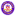Wilfrid Laurier University

Computational And Theoretical Aspects Of N-E.C. Graphs, Alexandru Costea

Theses and Dissertations (Comprehensive)

We consider graphs with the n-existentially closed adjacency property. For a positive integer n, a graph is n-existentially closed (or n-e.c.) if for all disjoint sets of vertices A and B with \AB\ = n (one of A or B can be empty), there is a vertex 2 not in AB joined to each vertex of A and no vertex of B. Although the n-e.c. property is straightforward to define, it is not obvious from the definition that graphs with the property exist. In 1963, Erdos and Rényi gave a non-explicit, randomized construction of ...

A New Nonlinear Classifier With A Penalized Signed Fuzzy Measure Using Effective Genetic Algorithm, 2010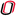University of Nebraska

A New Nonlinear Classifier With A Penalized Signed Fuzzy Measure Using Effective Genetic Algorithm, Julia Hua Fang, Maria L. Rizzo, Honggang Wang, Kimberly Espy, Zhenyuan Wang

Mathematics Faculty Publications

This paper proposes a new nonlinear classifier based on a generalized Choquet integral with signed fuzzy measures to enhance the classification accuracy and power by capturing all possible interactions among two or more attributes. This generalized approach was developed to address unsolved Choquet-integral classification issues such as allowing for flexible location of projection lines in n-dimensional space, automatic search for the least misclassification rate based on Choquet distance, and penalty on misclassified points. A special genetic algorithm is designed to implement this classification optimization with fast convergence. Both the numerical experiment and empirical case studies show that this generalized ...

2010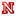University of Nebraska at Lincoln

Properties Of The Generalized Laplace Transform And Transport Partial Dynamic Equation On Time Scales, Chris R. Ahrendt

Dissertations, Theses, and Student Research Papers in Mathematics

In this dissertation, we first focus on the generalized Laplace transform on time scales. We prove several properties of the generalized exponential function which will allow us to explore some of the fundamental properties of the Laplace transform. We then give a description of the region in the complex plane for which the improper integral in the definition of the Laplace transform converges, and how this region is affected by the time scale in question. Conditions under which the Laplace transform of a power series can be computed term-by-term are given. We develop a formula for the Laplace transform for ...

2010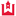Western Kentucky University

Derivatives Of The Dedekind Zeta Function Attached To A Complex Quadratic Field Extention, Nathan Salazar

Honors College Capstone Experience/Thesis Projects

The Riemann Zeta Function is a function of vital importance in the study of number theory and other branches of mathematics. This is primarily due to its intrinsic link with the prime numbers of the ring of integers. The value of the Riemann Zeta Function at 0 and the values of the first few derivatives at 0 have been determined by various mathematicians. Apostol obtained a closed expression for the nth derivative of the Riemann Zeta Function at 0 that generalized previously known results. For higher derivatives, his result is useful for numerical computations. The Dedekind Zeta Function is a ...

Structural Measurements For Enhanced Mav Flight, 2010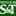Missouri University of Science and Technology

Structural Measurements For Enhanced Mav Flight, John R. Singler, Gregg Abate, Benjamin T. Dickinson

Mathematics and Statistics Faculty Research & Creative Works

Our sense of touch allows us to feel the forces in our limbs when we walk, swim, or hold our arms out the window of a moving car. We anticipate this sense is key in the locomotion of natural flyers. Inspired by the sense of touch, the overall goal of this research is to develop techniques for the estimation of aerodynamic loads from structural measurements for flight control applications. We submit a general algorithm for the direct estimation of distributed steady loads over bodies from embedded noisy deformation-based measurements. The estimation algorithm is applied to a linearly elastic membrane test ...

2010Missouri University of Science and Technology

High Accuracy Combination Method For Solving The Systems Of Nonlinear Volterra Integral And Integro-Differential Equations With Weakly Singular Kernels Of The Second Kind, Xiaoming He, Lu Pan, Tao Lü

Mathematics and Statistics Faculty Research & Creative Works

This paper presents a high accuracy combination algorithm for solving the systems of nonlinear Volterra integral and integro-differential equations with weakly singular kernels of the second kind. Two quadrature algorithms for solving the systems are discussed, which possess high accuracy order and the asymptotic expansion of the errors. By means of combination algorithm, we may obtain a numerical solution with higher accuracy order than the original two quadrature algorithms. Moreover an a posteriori error estimation for the algorithm is derived. Both of the theory and the numerical examples show that the algorithm is effective and saves storage capacity and computational ...

Gronwall-Ouiang-Type Integral Inequalities On Time Scales, 2010Missouri University of Science and Technology

Gronwall-Ouiang-Type Integral Inequalities On Time Scales, Ailian Liu, Martin Bohner

Mathematics and Statistics Faculty Research & Creative Works

We present several Gronwall-OuIang-type integral inequalities on time scales. Firstly, an OuIang inequality on time scales is discussed. Then we extend the Gronwall-type inequalities to multiple integrals. Some special cases of our results contain continuous Gronwall-type inequalities and their discrete analogues. Several examples are included to illustrate our results at the end.

The Hodrick-Prescott Filter: A Special Case Of Penalized Spline Smoothing, 2010Missouri University of Science and Technology

The Hodrick-Prescott Filter: A Special Case Of Penalized Spline Smoothing, Robert Paige L., A. A. Trindade

Mathematics and Statistics Faculty Research & Creative Works

We prove that the Hodrick-Prescott Filter (HPF), a commonly used method for smoothing econometric time series, is a special case of a linear penalized spline model with knots placed at all observed time points (except the first and last) and uncorrelated residuals. This equivalence then furnishes a rich variety of existing data-driven parameter estimation methods, particularly restricted maximum likelihood (REML) and generalized cross-validation (GCV). This has profound implications for users of HPF who have hitherto typically relied on subjective choice, rather than estimation, for the smoothing parameter. By viewing estimates as roots of an appropriate quadratic estimating equation, we also ...

2010Missouri University of Science and Technology

Incorporating Genome Annotation In The Statistical Analysis Of Genomic And Epigenomic Tiling Array Data, Gayla R. Olbricht

Mathematics and Statistics Faculty Research & Creative Works

"A wealth of information and technologies are currently available for the genomewide investigation of many types of biological phenomena. Genomic annotation databases provide information about the DNA sequence of a particular organism and give locations of different types of genomic elements, such as the exons and introns of genes. Microarrays are a powerful type of technology that make use of DNA sequence information to investigate different types of biological phenomena on a genome-wide level. Tiling arrays are a unique type of microarray that provide unbiased, highdensity coverage of a genomic region, making them well suited for many applications, such as ...

Nonlinear Model Reduction Using Group Proper Orthogonal Decomposition, 2010Missouri University of Science and Technology

Nonlinear Model Reduction Using Group Proper Orthogonal Decomposition, Benjamin T. Dickinson, John R. Singler

Mathematics and Statistics Faculty Research & Creative Works

We propose a new method to reduce the cost of computing nonlinear terms in projec- tion based reduced order models with global basis functions. We develop this method by extending ideas from the group nite element (GFE) method to proper orthogonal decomposition (POD) and call it the group POD method. Here, a scalar two-dimensional Burgers' equation is used as a model problem for the group POD method. Numerical results show that group POD models of Burgers' equation are as accurate and are computationally more e cient than standard POD models of Burgers' equation.

2010Missouri University of Science and Technology

Optimality Of Balanced Proper Orthogonal Decomposition For Data Reconstruction, John R. Singler

Mathematics and Statistics Faculty Research & Creative Works

Proper orthogonal decomposition (POD) finds an orthonormal basis yielding an optimal reconstruction of a given dataset. We consider an optimal data reconstruction problem for two general datasets related to balanced POD, which is an algorithm for balanced truncation model reduction for linear systems. We consider balanced POD outside of the linear systems framework, and prove that it solves the optimal data reconstruction problem. the theoretical result is illustrated with an example.

Weyl-Titchmarsh Theory For Hamiltonian Dynamic Systems, 2010Missouri University of Science and Technology

Weyl-Titchmarsh Theory For Hamiltonian Dynamic Systems, Shurong Sun, Shaozhu Chen, Martin Bohner

Mathematics and Statistics Faculty Research & Creative Works

We establish the Weyl-Titchmarsh theory for singular linear Hamiltonian dynamic systems on a time scale T , which allows one to treat both continuous and discrete linear Hamiltonian systems as special cases for T= ℝ and T= ℤ within one theory and to explain the discrepancies between these two theories. This paper extends the Weyl-Titchmarsh theory and provides a foundation for studying spectral theory of Hamiltonian dynamic systems. These investigations are part of a larger program which includes the following: (i) M(λ) theory for singular Hamiltonian systems, (ii) on the spectrum of Hamiltonian systems, (iii) on boundary value problems for ...

Balanced Pod Algorithm For Robust Control Design For Linear Distributed Parameter Systems, 2010Missouri University of Science and Technology

Balanced Pod Algorithm For Robust Control Design For Linear Distributed Parameter Systems, John R. Singler, Belinda A. Batten

Mathematics and Statistics Faculty Research & Creative Works

A mathematical model of a physical system is never perfect; therefore, robust control laws are necessary for guaranteed stabilization of the nominal model and also "nearby" systems, including hopefully the actual physical system. We consider the computation of a robust control law for large-scale finite dimensional linear systems and a class of linear distributed parameter systems. The controller is robust with respect to left coprime factor perturbations of the nominal system. We present an algorithm based on balanced proper orthogonal decomposition to compute the nonstandard features of this robust control law. Numerical results are presented for a convection diffusion partial ...

Computational Issues In Sensitivity Analysis For 1d Interface Problems, 2010Missouri University of Science and Technology

Computational Issues In Sensitivity Analysis For 1d Interface Problems, L. G. Davis, John R. Singler

Mathematics and Statistics Faculty Research & Creative Works

This paper is concerned with the construction of accurate and e cient computational algorithms for the numerical approximation of sensitivities with respect to a parameter dependent interface location. Motivated by sensitivity analysis with respect to piezoelectric actuator placement on an